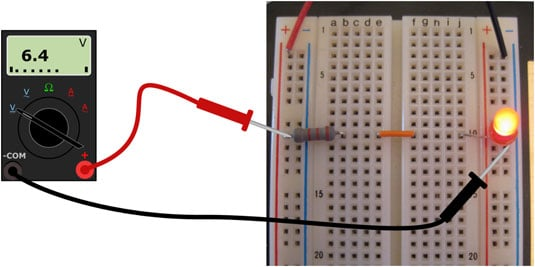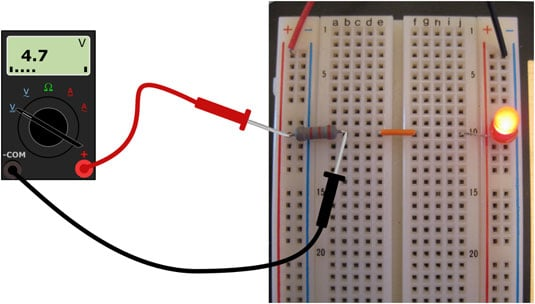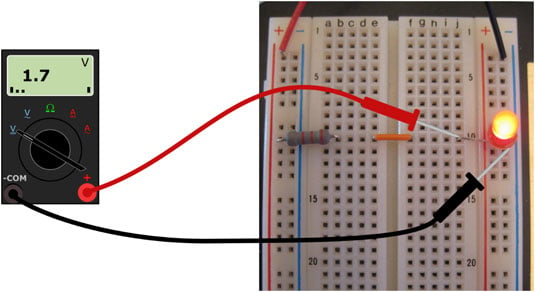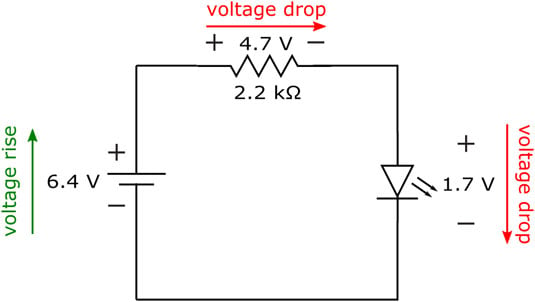##### Electronics For DummiesYou can use your multimeter to measure the voltage across the battery pack, the resistor, and the LED in a circuit. Note that the connection points between components are the same whether you built the circuit using a breadboard or alligator clips.

The red lead of your multimeter should be at a higher voltage than the black lead, so take care to orient the probes as described. Set your multimeter to measure DC voltage and get ready to take some measurements!

First, measure the voltage supplied to the circuit by the battery pack. Connect the positive (red) multimeter lead to the point where the positive (red lead) side of the battery pack connects to the resistor, and the negative (black) multimeter lead to the point where the negative (black lead) side of the battery pack connects to the LED. See the following figure. Do you get a voltage reading that is close to the nominal supply voltage of 6 V? (Fresh batteries may supply more than 6 V; old batteries usually supply less than 6 V.)Measure the voltage supplied by the battery pack.

Next, measure the voltage across the resistor. Connect the positive (red) multimeter lead to the point where the resistor connects with the positive side of the battery pack, and the negative (black) multimeter lead to the other side of the resistor. See the following figure. Your voltage reading should be close to the one that appears on the multimeter in the figure.Measure the voltage across the resistor.

Finally, measure the voltage across the LED. Place the red multimeter lead to the point where the LED connects with the resistor, and the black multimeter lead to the point where the LED connects to the negative side of the battery pack. See the following figure. Was your voltage reading close to the one in the figure?Measure the voltage across the LED.

The measurements show that in this circuit, the battery pack is supplying 6.4 volts, and that 4.7 volts are dropped across the resistor and 1.7 volts are dropped across the LED. It's not a coincidence that the sum of the voltage drops across the resistor and the LED is equal to the voltage supplied by the battery pack:

4.7 V + 1.7 V = 6.4 V

A give-and-take relationship is going on in this circuit: Voltage is the push the battery gives to get current moving, and energy from that push is absorbed when current moves through the resistor and the LED. As current flows through the resistor and the LED, voltage drops across each of those components. The resistor and the LED are using up energy supplied by the force (voltage) that pushes the current through them.

You can rearrange the preceding voltage equation to show that the resistor and the LED are dropping voltage as they use up the energy supplied by the battery:

64 V – 4.7 V – 1.7 V = 0

When you drop voltage across a resistor, an LED, or another component, the voltage is more positive at the point where the current enters the component than it is at the point where the current exits the component. Voltage is a relative measurement because it's the force that results from a difference in charge from one point to another.

The voltage supplied by a battery represents the difference in charge from the positive terminal to the negative terminal, and that difference in charge has the potential to move current through a circuit; the circuit, in turn, absorbs the energy generated by that force as the current flows, which drops the voltage. No wonder voltage is sometimes called voltage drop, potential difference, or potential drop.

The important thing to note here is that as you travel around a DC circuit, you gain voltage going from the negative terminal of the battery to the positive terminal (that's known as a voltage rise), and you lose, or drop, voltage as you continue in the same direction across circuit components. (See the following figure.) By the time you get back to the negative terminal of the battery, all the battery voltage has been dropped and you're back to 0 volts.The voltage supplied by the battery is dropped across the resistor and the LED.

With all circuits (whether AC or DC), if you start at any point in the circuit, and add the voltage rises and drops going around the circuit, you get zero volts. In other words, the net sum of the voltage rises and drops in voltage around a circuit is zero. (This rule is known as Kirchhoff's Voltage Law. Kirchhoff is pronounced "keer-cough.")

Keep in mind that these voltage drops have a physical meaning. The electrical energy supplied by the battery is absorbed by the resistor and the LED. The battery will keep supplying electrical energy, and the resistor and LED will keep absorbing that energy, until the battery dies (runs out of energy). That happens when all the chemicals inside the battery have been consumed in the chemical reactions that produced the positive and negative charges. In effect, all the chemical energy supplied by the battery has been converted into electrical energy — and absorbed by the circuit.

One of the fundamental laws of physics is that energy cannot be created or destroyed; it can only change form. You witness this law in action with the simple battery-driven LED circuit: Chemical energy is converted to electrical energy, which is converted to heat and light energy, which — well, you get the idea.